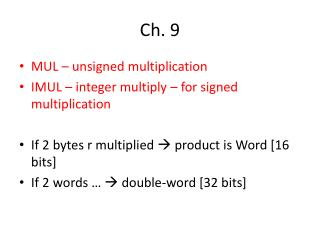DownloadDownload PresentationCh. 9

# Ch. 9

Download Presentation## Ch. 9

- - - - - - - - - - - - - - - - - - - - - - - - - - - E N D - - - - - - - - - - - - - - - - - - - - - - - - - - -
##### Presentation Transcript

1. Ch. 9 • MUL – unsigned multiplication • IMUL – integer multiply – for signed multiplication • If 2 bytes r multiplied  product is Word [16 bits] • If 2 words …  double-word [32 bits]

2. Byte form – • MUL source ;source= register, mem; not const. • One no. is in ‘source’ • Another no. is in AL • Product is in AX

3. Word form • Source ; 16-bit reg/mem – not const. • AX • MSB in DX, LSB in AX [DX:AX]

4. Q. A = 5xA – 12xB A, B = word variables. No overflow. MOV AX, 5 IMUL A ;AX=5xA MOVA,AX ;A=5A MOV AX,12 ;AX=12 IMUL B ;AX=12B SUB A,AX ;A=5A – 12B

5. Q. Factorial that will compute N!

6. DIV, IDIV • DIV – divide – unsigned division • IDIV – integer divide • DIV divisor Byte form: • Divisor  8-bt reg/mem • Dividend  16-bit in AX • After division, Quotient  8-bit in AL • …, Remainder  8-bit in AH 15 ÷ 3 = 5, 3 is the divisor

7. Word form: • Divisor  16-bit reg/mem • Dividend  32-bit in DX:AX • After division, Quotient  16-bit in AX • …, Remainder  16-bit in DX

8. Divide overflow

9. Ch. 10 • 1-D array • DUP – to define arrays whose elements share a common initial value • E.g., GAMMA DW 100 DUP (0) • Sets up an array of 100 words, with each entry – initialized to 0. GAMMA DW 100 DUP (?)  Sets up an array of 100 words, with each entry – UN-initialized.

10. DUPs may be nested…